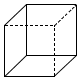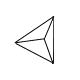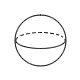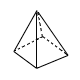Create a new printable
Answer key also includes questions
Answer key only gives the answers
No answer keyMath Worksheets

Sample - Click above to make a new math worksheet (PDF).
 Name _____________________________Date ___________________
Solid Shapes
Circle the name of the shape. Write how many faces, vertices, and edges it has.
A face is a flat surface.  An edge is where two faces meet.  A vertex is where three faces meet.
Hint: Remember that a face needs to be flat.

1.cone cube
 pyramid sphere
 Faces:Edges:Vertices:2.cylinder sphere
 pyramid rectangular prism
 Faces:Edges:Vertices:3.cone sphere
 pyramid cylinder
 Faces:Edges:Vertices:4.cone cube
 pyramid cylinder
 Faces:Edges:Vertices:5 * This is a pre-made sheet.Use the link at the top of the page for a printable page.

 Answer Key

Sample
This is only a sample worksheet.

Create a new printable
Answer key also includes questions
Answer key only gives the answers
No answer keyMath Worksheets